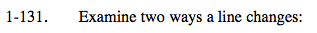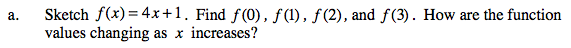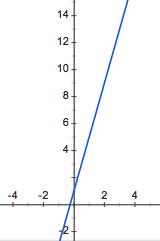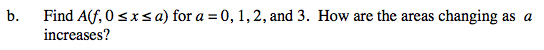### Home > CALC > Chapter 1 > Lesson 1.3.3 > Problem1-131

1-131.
1. Examine two ways a line changes: Homework Help ✎

1. Sketch f(x) = 4x + 1. Find f(0), f(l), f(2), and f(3). How are the function values changing as x increases?

2. Find A(f, 0 ≤ xa) for a = 0, 1,2, and 3. How are the areas changing as a increases?Compute the finite differences (that is, find the difference in y-values as x increases) and look for a pattern.Use geometry to find the area of the trapezoid between x = 0 and x = 2 for each value of a. Keep your results organized.

Is the difference between the areas growing constantly or linearly? If not, how else could it be growing, and by what pattern each time?